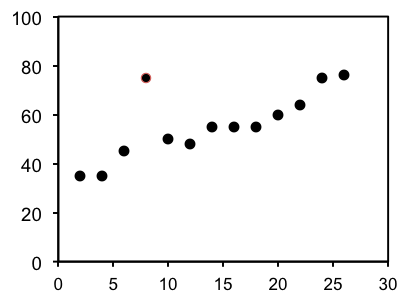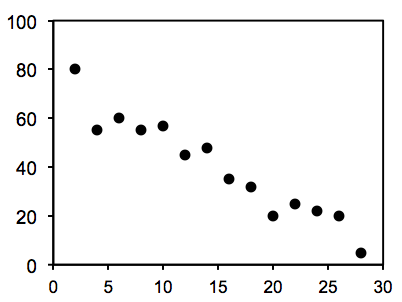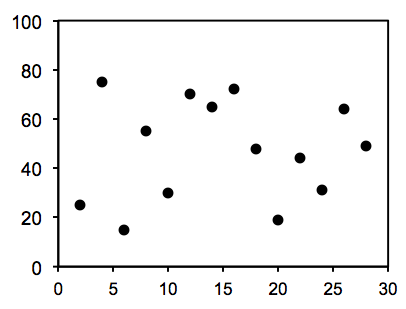## Information

How do you show a correlation even exists in the first place, in order to provide the first step in establishing a causal relationship? Usually you plot the two variables you think might be correlated. If two variables are correlated, plotting them on a scatter plot should produce a straight-line relationship.

Statisticians have developed equations to quantitate how well data fit onto lines with defined equations. The line you most often see data fit to in graphs is a straight line. A straight line has the equation y=mx+b, where m is the slope of the line and b is the y-intercept. Data rarely fit perfectly on a line, so the equations statisticians have developed report a correlation coefficient for the fit of the data to the line. For various reasons, correlation coefficients are also known as “r values.”

If data fit perfectly on a line, then the correlation coefficient will be either r = 1.0 or r = -1.0. You cannot have anything better than a perfect fit, so 1.0 is the largest positive r value possible and -1.0 is the largest negative r value possible.

When r = 1.0, there is a perfect positive correlation. That means that when the first variable increases, the second variable also increases by the exact same proportion (if the first variable increases two-fold, the second variable increases two-fold.) And when the first variable decreases, the second variable also decreases by the exact same proportion. With a positive correlation, the two variables move in the same direction.

When r = -1.0, there is a perfect negative correlation. That means that when the first variable increases, the second variable decreases by the exact same proportion (if the first variable increases two-fold, the second variable decreases two-fold.) And when the first variable decreases, the second variable increases by the exact same proportion. With a negative correlation, the two variables move in opposite directions.

If there is no relationship between the two variables being plotted—if the one variable has no consistent relationship with the other—then the data is said to have a correlation coefficient of r = 0.0, and no correlation.

If a scientific graph has a line drawn through the data, it should always report the correlation coefficient for that line, so that the readers can see for themselves how well the data fit the line. The closer the r value is to 1.0 or -1.0, the more convincing the fit. The closer the r value is to 0.0, the greater the likelihood that the two variables have no relationship with each other and no effect on one another.

The following three graphs represent positive correlations that show a perfect fit (r = 1.0), a strong fit (r = 0.90) and a non-existing fit (r = 0.0).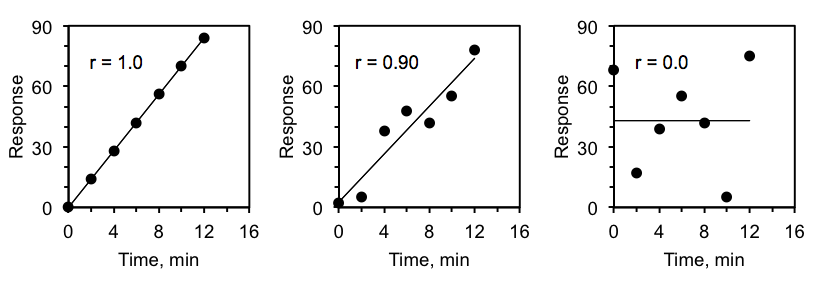Figure 2-2. Three graphs illustrating positive correlations with three degrees of fit of data to a straight line as indicated by their correlation coefficients’ r values.

Negative correlations are also known as indirect correlations. Whatever it is called, as is illustrated in Figure 2.2 below, a negative correlation will show one variable decreasing as the other increases. The stronger the negative correlation, the closer the correlation coefficient, r, is to -1.0. When the correlation coefficient is 0.0 or close to 0.0, there is essentially no correlation.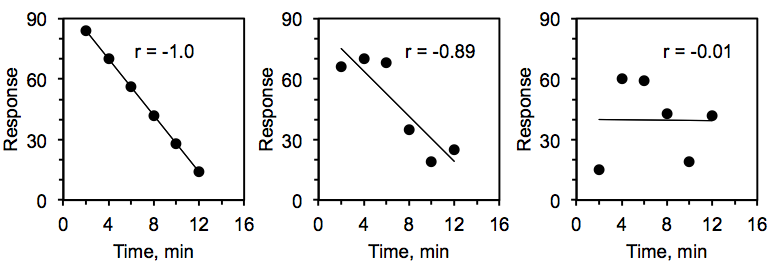Figure 2-3. Three graphs illustrating negative correlations with three degrees of fit of data to a straight line as indicated by their correlation coefficient’s r values.

In some graphs, rather than report correlation coefficients, or r values, the researchers report coefficients of determination, or r2, values. There is a distinction between the two in what they literally mean, but the distinction between r values and r2 values is beyond the scope of this lab. For most practical purposes, you can assume the r2 value reveals essentially the same information as the r value. It tells you how well the graphed data fit a straight line.

The major difference is that r2 values are always positive, regardless of whether the data are directly correlated or indirectly correlated. As a result, r2 values are always in the range $0.0\leq{r}^{2}\leq1.0$. As with r values, if r2 = 0.0, then there is no correlation between the two variables, and if r2 = 1.0 they are perfectly correlated. Positive and negative correlations both give r2 values of 1.0 if they are perfectly correlated.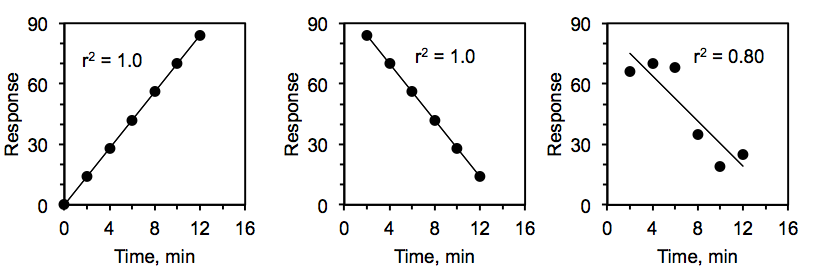Figure 2-4. Three graphs illustrating how r2 values indicate how well straight lines fit data.

You don’t need to know the equations for how to calculate correlation coefficients or coefficients of determination for this course. Calculators and graphing programs like Excel will calculate them for you. You just need to know how to interpret them.

In general, the closer a correlation coefficient (the r value) is to 1.0 in the case of positive correlations, or the closer it is to -1.0 in the case of negative correlations, the stronger the correlation is said to be. (Remember, if the coefficient of determination, or r2 value, is reported, both positive and negative correlations will have positive r2 values, and the closer that value is to 1.0, the stronger the correlation will be.)

There is no hard and fast rule as to when a correlation coefficient to close enough to 0.0 to rule the correlation as non-existent, but if the numerical value is less than 0.3 most researchers will conclude that the correlation is too weak to consider significant.

### Lab 2 Exercises 2.6

1. Use the following guidelines to estimate the best-fitting straight line through the data in the three graphs below and then draw in the best-fitting straight line with a ruler. Next to each graph, indicate what kind of correlation, in general, you are looking at.
• The best-fitting straight line will have the maximal number of data points as close as possible to the line.
• If there are a few data points that are far away from the line, that is okay as long as most of the other data points are as close to the line as possible.
• Ideally there should be as many data points above the line as there are below it.
• It is better to have none of the data points actually on the line but most of them as close as possible than to have a few points on the line but the rest of the points farther away than they would be in the line were just moved a bit.
• Best-fitting lines do not have to go through (0,0) if the origin does not fit the rest of the data.
Graph Type of Correlation